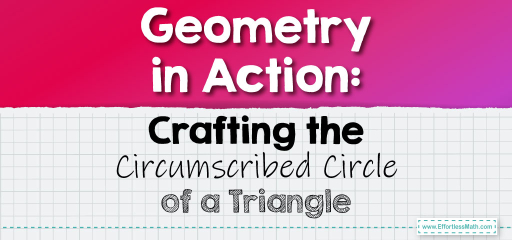# Geometry in Action: Crafting the Circumscribed Circle of a Triangle

In geometry, every triangle has a unique circumscribed circle, also known as its circumcircle, that perfectly touches all its three vertices. The center of this circle, termed the circumcenter, is equidistant from the triangle's vertices. Constructing the circumcircle involves a blend of fundamental geometric concepts and precision. This guide elucidates the process in depth.## Step-by-step Guide: The Circumscribed Circle of a Triangle

Tools Required:

• Straightedge or Ruler: Essential for drawing straight lines and segments.
• Compass: Critical for creating arcs and circles.
• Pencil: To mark points and draw figures.

Procedure:

1. Base Triangle: Start by sketching triangle $$ABC$$. This will serve as the foundation for our subsequent constructions.

2. Perpendicular Bisector for $$AB$$:
– Position the compass point on vertex $$A$$, and set its width slightly more than half the length of segment $$AB$$.
– Draw arcs above and below segment $$AB$$.
– Without adjusting the compass width, place the compass point on vertex $$B$$ and draw arcs that intersect the previous arcs.
– Using the straightedge, connect these intersections to create the perpendicular bisector of $$AB$$.

3. Perpendicular Bisector for $$AC$$:
– Repeat the process for segment $$AC$$, drawing arcs centered at $$A$$ and $$C$$.
– The line formed by connecting their intersections is the perpendicular bisector for $$AC$$.

4. Finding the Circumcenter:
– The circumcenter, $$O$$, is the point where the perpendicular bisectors of $$AB$$ and $$AC$$ intersect.
– It holds a special property: the distances from $$O$$ to $$A$$, $$B$$, and $$C$$ are all equal.

5. Drawing the Circumscribed Circle:
– Adjust your compass to the distance between circumcenter $$O$$ and any triangle vertex, say $$A$$.
– With the compass point at $$O$$, draw a circle. This circle, which touches all vertices of $$ABC$$, is the triangle’s circumcircle.

### Examples

Example 1:
You are designing a logo, and you want to emphasize the unity of three elements represented by a triangle. However, you’d like to encircle this triangle with a perfect circle to symbolize completeness.

Solution:
Using the steps above, first sketch your triangle. Then, construct the circumcircle around it, ensuring both geometric figures are in harmony and proportion, achieving the desired symbolism.

Example 2:
Does the circumcenter always lie inside the triangle?

Solution:
No. For an acute triangle, the circumcenter lies inside. For a right triangle, it lies on the hypotenuse. However, for an obtuse triangle, the circumcenter is located outside the triangle.

### Practice Questions:

1. For an obtuse triangle, where does the circumcenter lie?
2. Construct the circumcircle for a triangle with given side lengths using a ruler and compass. How would the process vary for different triangle types?
3. If a triangle’s sides are given, can we determine the circumradius (radius of the circumcircle) without constructing it?

1. For an obtuse triangle, the circumcenter lies outside the triangle.
2. The core steps remain consistent, but the location of the circumcenter varies. For acute triangles, it’s inside; for right triangles, it’s on the hypotenuse; for obtuse triangles, it’s outside.
3. Yes, using the triangle’s side lengths and semi-perimeter, the circumradius $$R$$ can be determined by the formula:
$$R = \frac{abc}{4K}$$
Where $$a$$, $$b$$, and $$c$$ are the triangle’s sides, and $$K$$ is its area.

### What people say about "Geometry in Action: Crafting the Circumscribed Circle of a Triangle - Effortless Math: We Help Students Learn to LOVE Mathematics"?

No one replied yet.

X
30% OFF

Limited time only!

Save Over 30%

SAVE $5 It was$16.99 now it is \$11.99